Interface to the PPC (posterior predictive checking) module in the bayesplot package, providing various plots comparing the observed outcome variable $$y$$ to simulated datasets $$y^{rep}$$ from the posterior predictive distribution. The pp_check method for stanreg-objects prepares the arguments required for the specified bayesplot PPC plotting function and then calls that function. It is also straightforward to use the functions from the bayesplot package directly rather than via the pp_check method. Examples of both are given below.

# S3 method for stanreg
pp_check(object, plotfun = "dens_overlay", nreps = NULL, seed = NULL, ...)

## Arguments

object A fitted model object returned by one of the rstanarm modeling functions. See stanreg-objects. A character string naming the bayesplot PPC function to use. The default is to call ppc_dens_overlay. plotfun can be specified either as the full name of a bayesplot plotting function (e.g. "ppc_hist") or can be abbreviated to the part of the name following the "ppc_" prefix (e.g. "hist"). To get the names of all available PPC functions see available_ppc. The number of $$y^{rep}$$ datasets to generate from the posterior predictive distribution and show in the plots. The default depends on plotfun. For functions that plot each yrep dataset separately (e.g. ppc_hist), nreps defaults to a small value to make the plots readable. For functions that overlay many yrep datasets (e.g., ppc_dens_overlay) a larger number is used by default, and for other functions (e.g. ppc_stat) the default is to set nreps equal to the posterior sample size. An optional seed to pass to posterior_predict. Additonal arguments passed to the bayesplot function called. For many plotting functions ... is optional, however for functions that require a group or x argument, these arguments should be specified in .... If specifying group and/or x, they can be provided as either strings naming variables (in which case they are searched for in the model frame) or as vectors containing the actual values of the variables. See the Examples section, below.

## Value

pp_check returns a ggplot object that can be further customized using the ggplot2 package.

## Note

For binomial data, plots of $$y$$ and $$y^{rep}$$ show the proportion of 'successes' rather than the raw count. Also for binomial models see ppc_error_binned for binned residual plots.

Gelman, A., Carlin, J. B., Stern, H. S., Dunson, D. B., Vehtari, A., and Rubin, D. B. (2013). Bayesian Data Analysis. Chapman & Hall/CRC Press, London, third edition. (Ch. 6)

Gabry, J. , Simpson, D. , Vehtari, A. , Betancourt, M. and Gelman, A. (2019), Visualization in Bayesian workflow. J. R. Stat. Soc. A, 182: 389-402. doi:10.1111/rssa.12378, (journal version, arXiv preprint, code on GitHub)

• The vignettes in the bayesplot package for many examples. Examples of posterior predictive checks can also be found in the rstanarm vignettes and demos.

• PPC-overview (bayesplot) for links to the documentation for all the available plotting functions.

• posterior_predict for drawing from the posterior predictive distribution.

• color_scheme_set to change the color scheme of the plots.

## Examples

fit <- stan_glmer(
mpg ~ wt + am + (1|cyl),
data = mtcars,
iter = 400, # iter and chains small just to keep example quick
chains = 2,
refresh = 0
)#> Warning: Bulk Effective Samples Size (ESS) is too low, indicating posterior means and medians may be unreliable.
#> Running the chains for more iterations may help. See
#> http://mc-stan.org/misc/warnings.html#bulk-ess#> Warning: Tail Effective Samples Size (ESS) is too low, indicating posterior variances and tail quantiles may be unreliable.
#> Running the chains for more iterations may help. See
#> http://mc-stan.org/misc/warnings.html#tail-ess
# Compare distribution of y to distributions of multiple yrep datasets
pp_check(fit)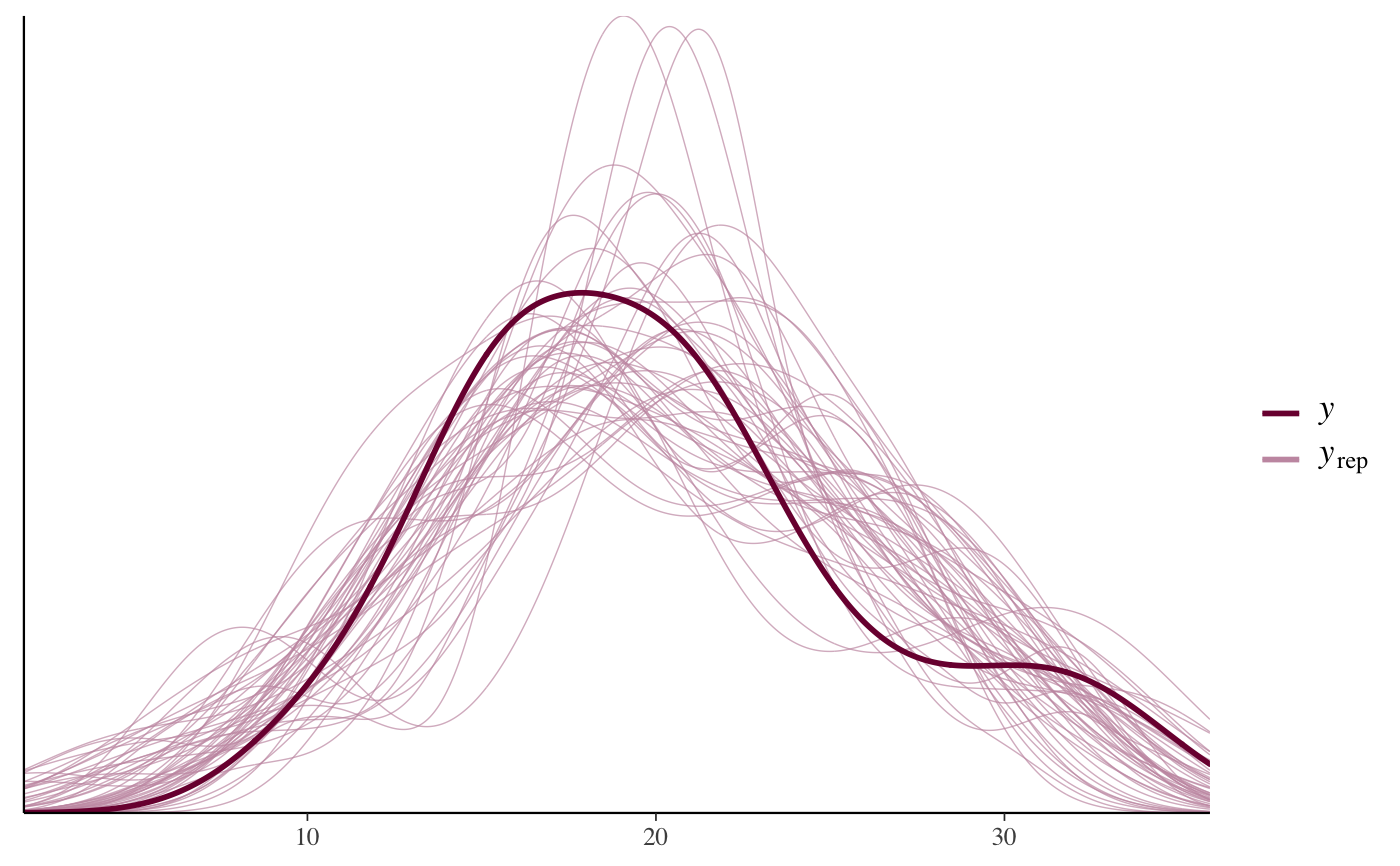pp_check(fit, plotfun = "boxplot", nreps = 10, notch = FALSE)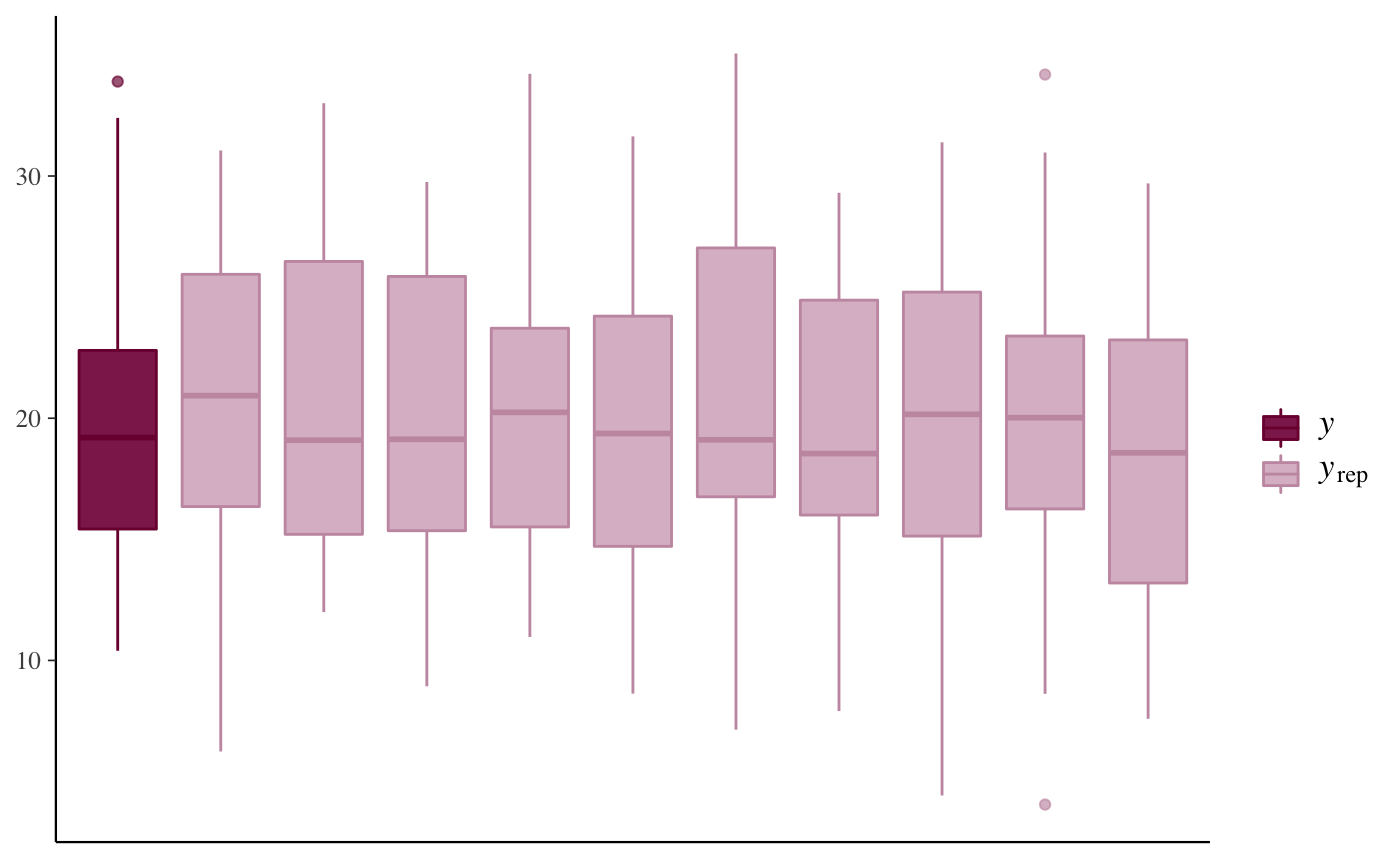pp_check(fit, plotfun = "hist", nreps = 3)#> stat_bin() using bins = 30. Pick better value with binwidth.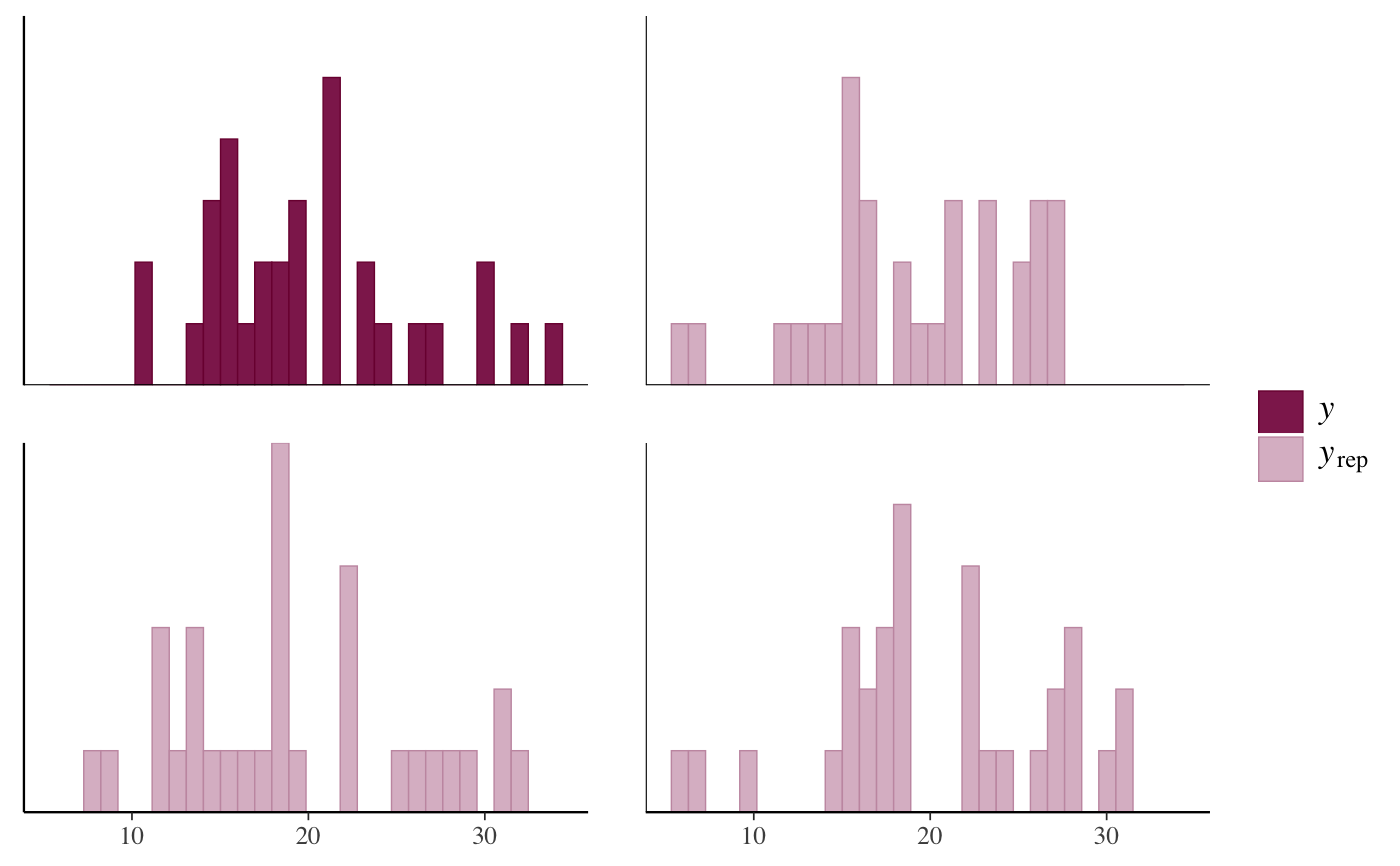# \donttest{
# Same plot (up to RNG noise) using bayesplot package directly
bayesplot::ppc_hist(y = mtcars$mpg, yrep = posterior_predict(fit, draws = 3))#> stat_bin() using bins = 30. Pick better value with binwidth.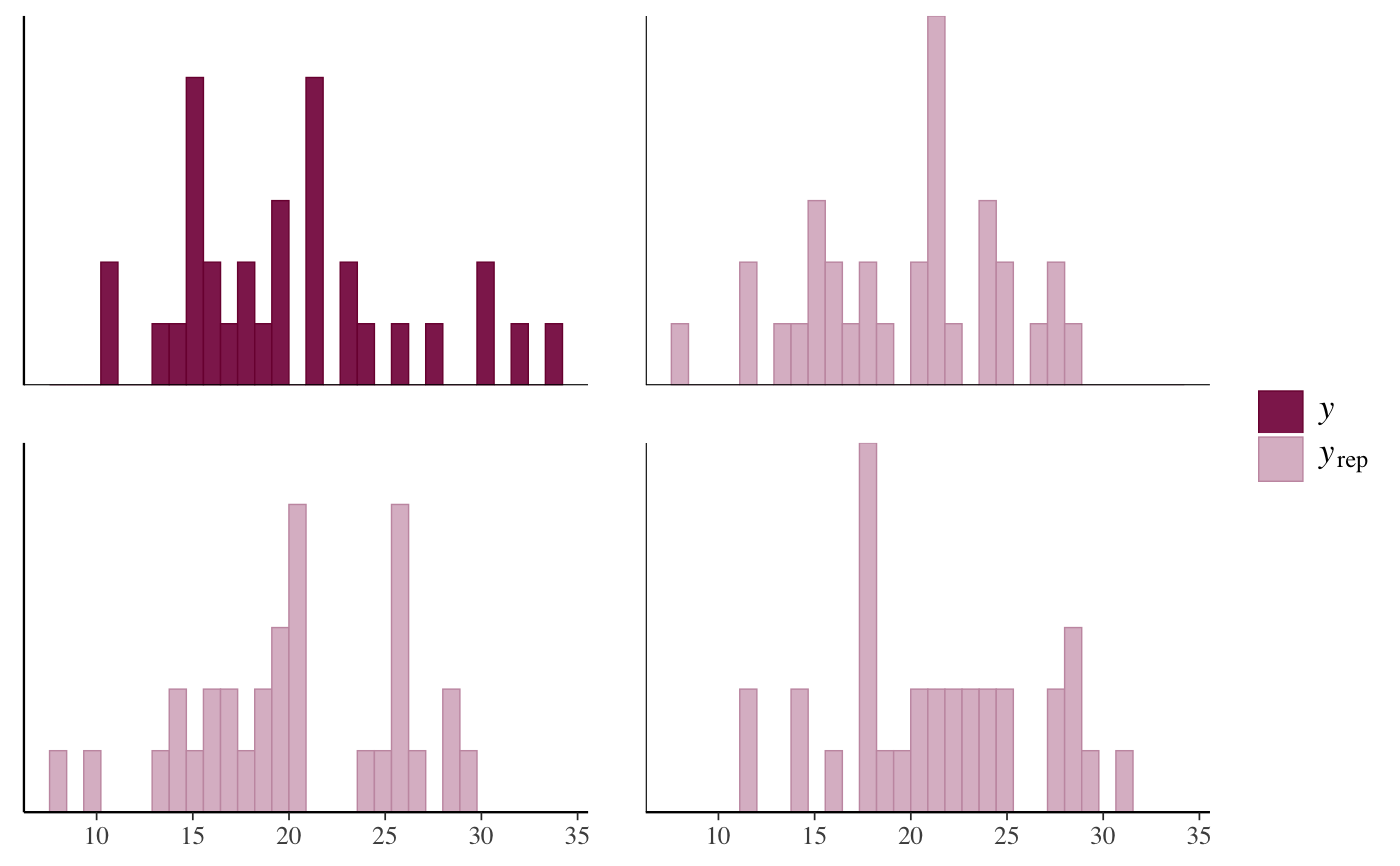# Check histograms of test statistics by level of grouping variable 'cyl' pp_check(fit, plotfun = "stat_grouped", stat = "median", group = "cyl")#> stat_bin() using bins = 30. Pick better value with binwidth.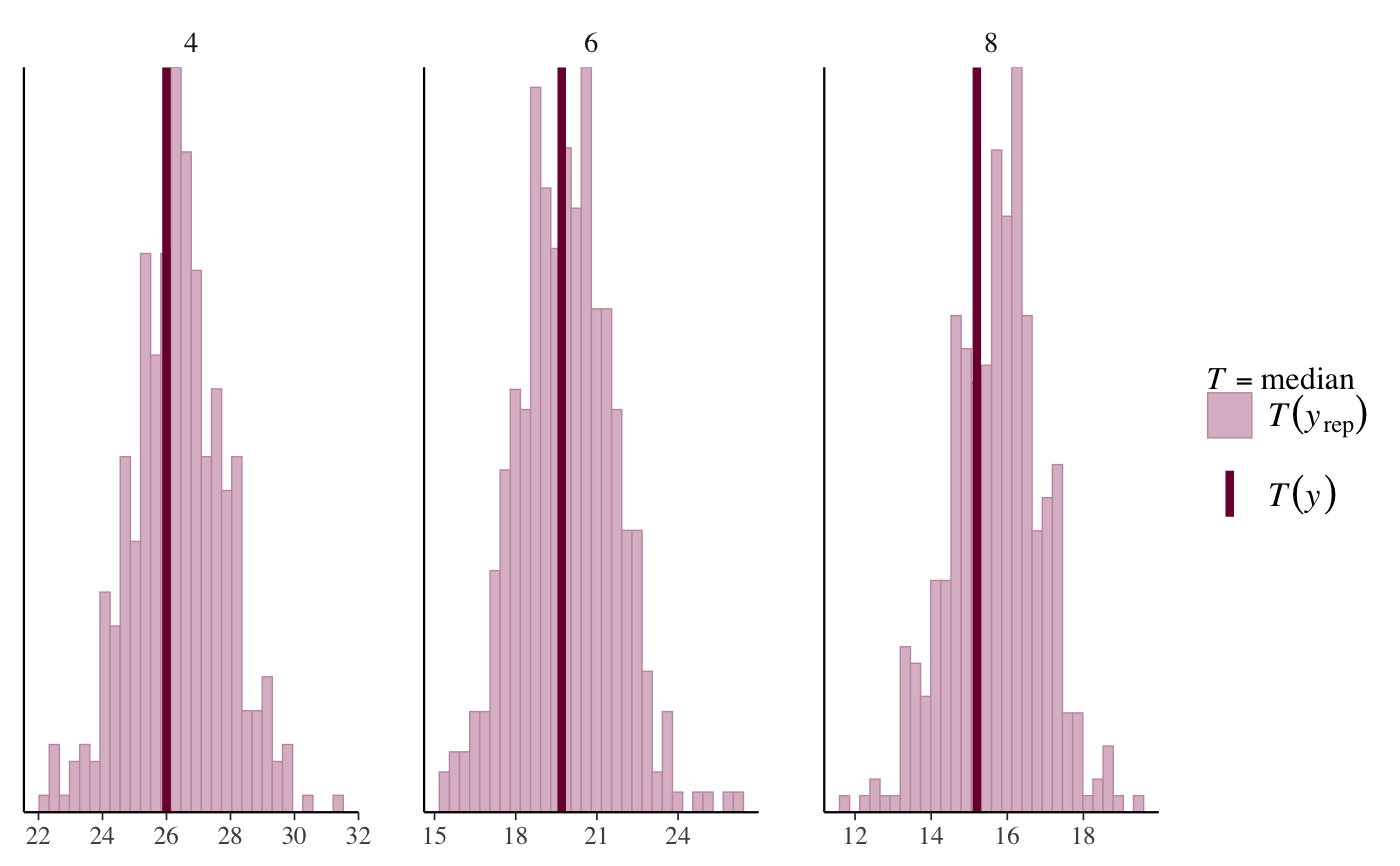# Defining a custom test statistic q25 <- function(y) quantile(y, probs = 0.25) pp_check(fit, plotfun = "stat_grouped", stat = "q25", group = "cyl")#> Error in get(as.character(FUN), mode = "function", envir = envir): object 'q25' of mode 'function' was not found # Scatterplot of two test statistics pp_check(fit, plotfun = "stat_2d", stat = c("mean", "sd"))# Scatterplot of y vs. average yrep pp_check(fit, plotfun = "scatter_avg") # y vs. average yrep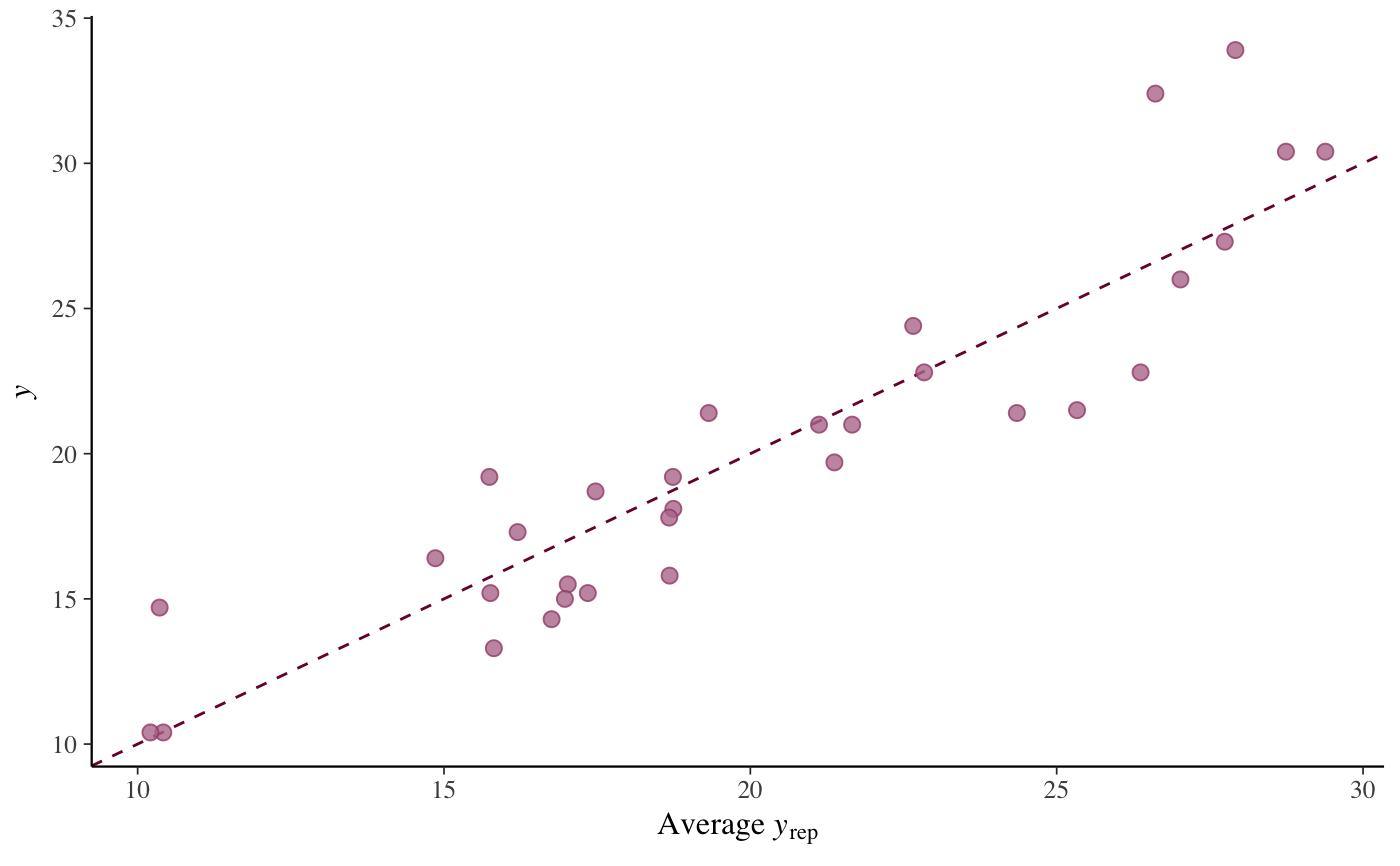# Same plot (up to RNG noise) using bayesplot package directly bayesplot::ppc_scatter_avg(y = mtcars$mpg, yrep = posterior_predict(fit))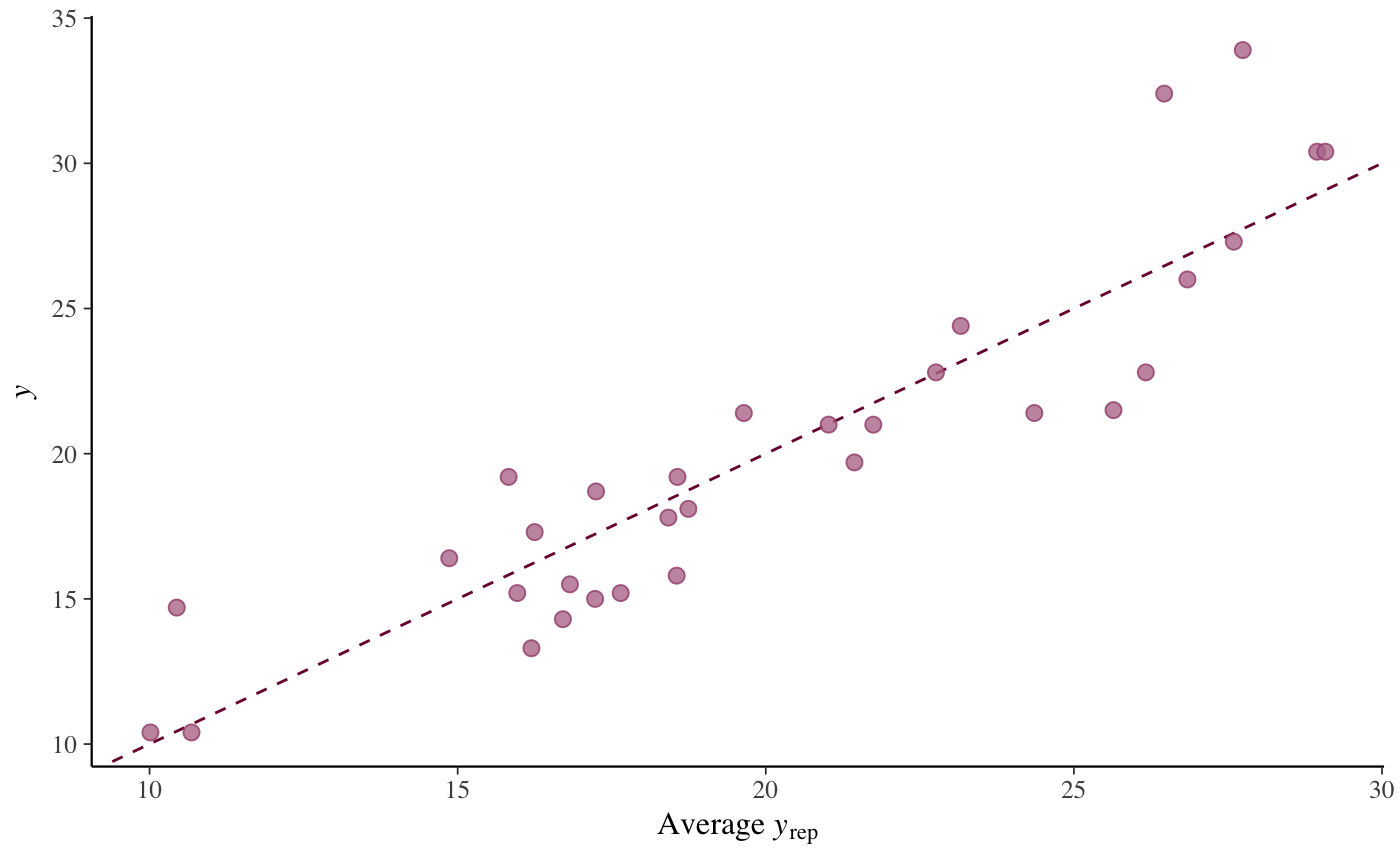# Scatterplots of y vs. several individual yrep datasets
pp_check(fit, plotfun = "scatter", nreps = 3)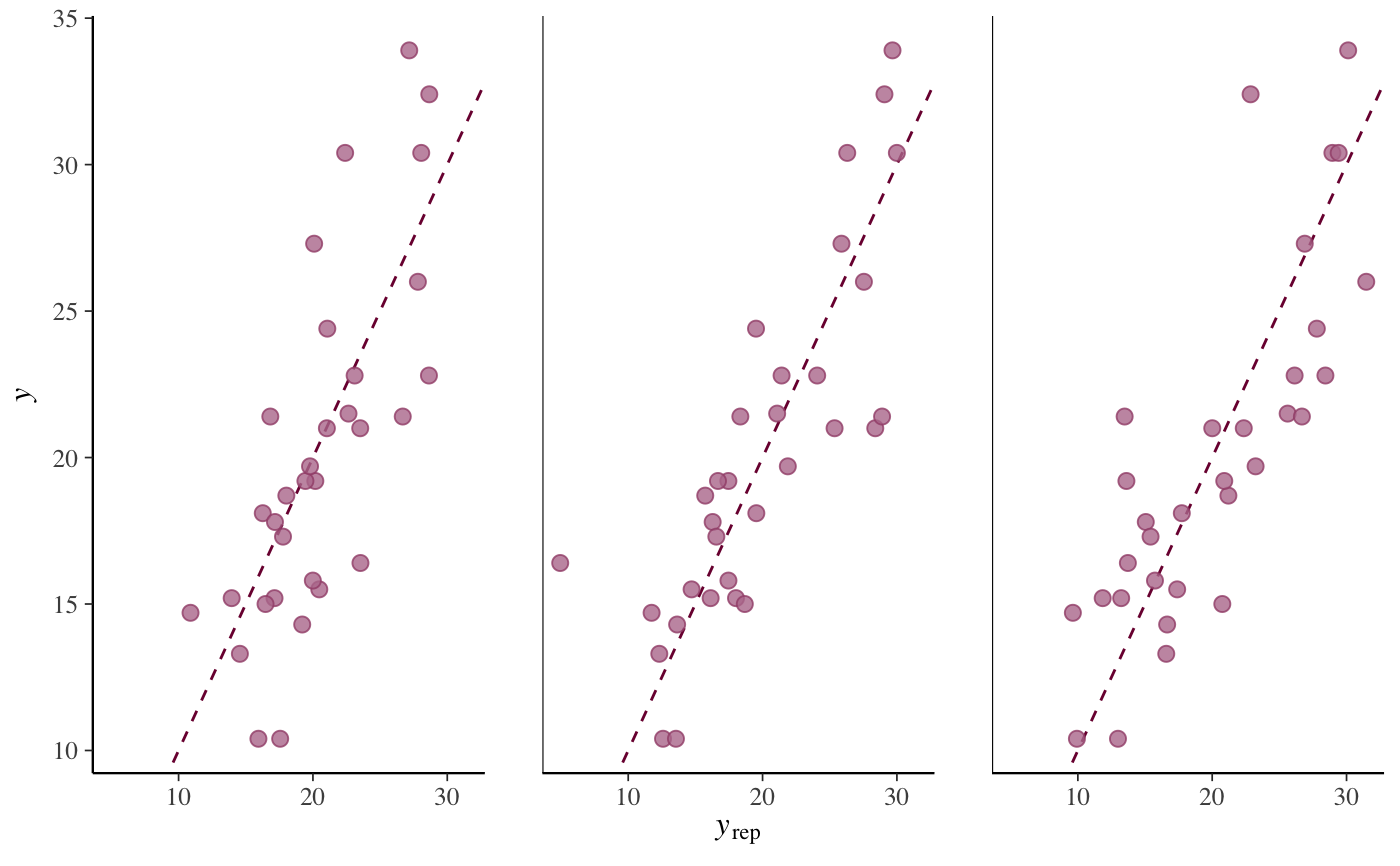# Same plot (up to RNG noise) using bayesplot package directly
bayesplot::ppc_scatter(y = mtcars$mpg, yrep = posterior_predict(fit, draws = 3))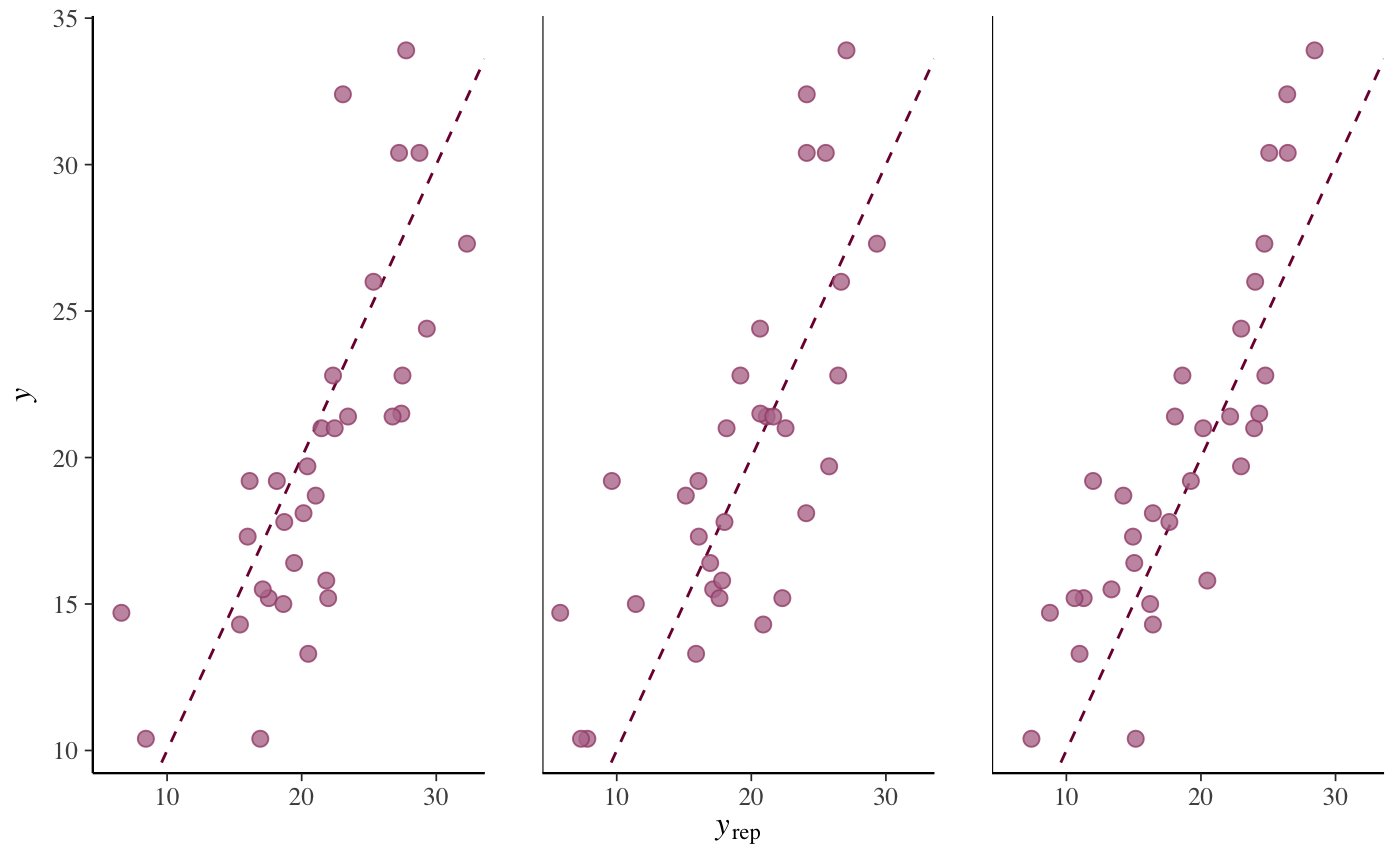# yrep intervals with y points overlaid # by default 1:length(y) used on x-axis but can also specify an x variable pp_check(fit, plotfun = "intervals")#> 'x' not specified in '...'. Using x=1:length(y).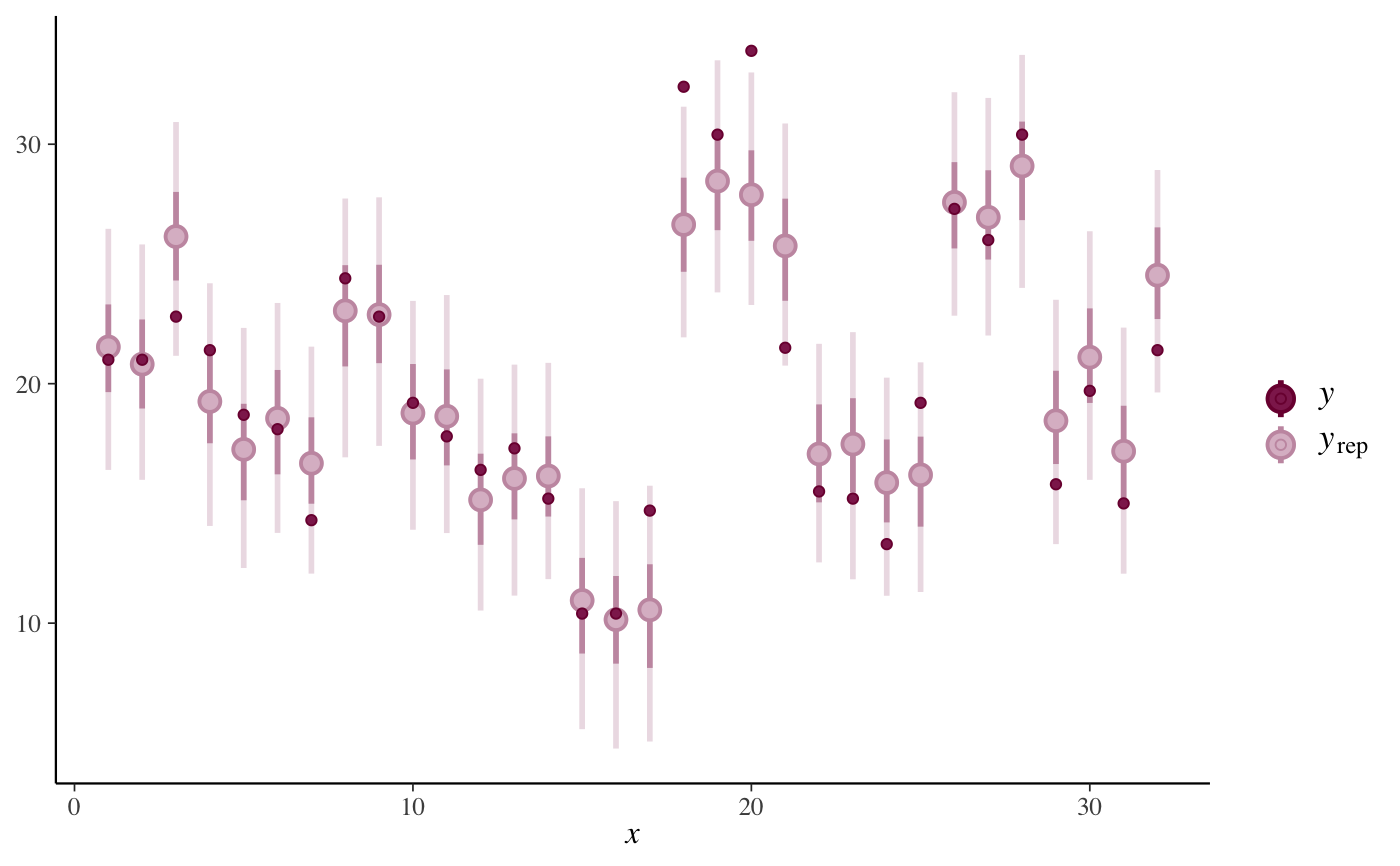pp_check(fit, plotfun = "intervals", x = "wt") + ggplot2::xlab("wt")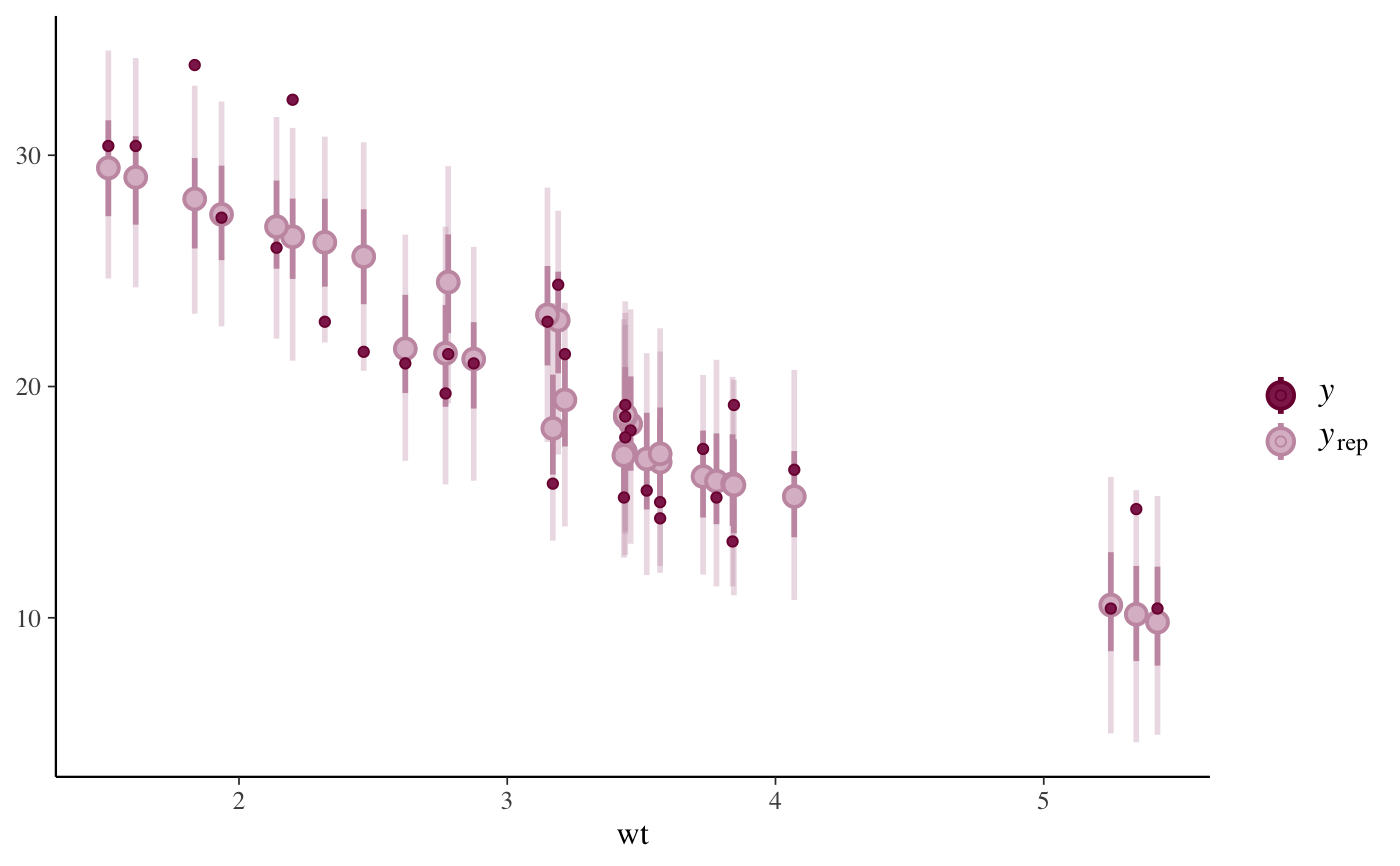# Same plot (up to RNG noise) using bayesplot package directly bayesplot::ppc_intervals(y = mtcars$mpg, yrep = posterior_predict(fit),
x = mtcars$wt) + ggplot2::xlab("wt")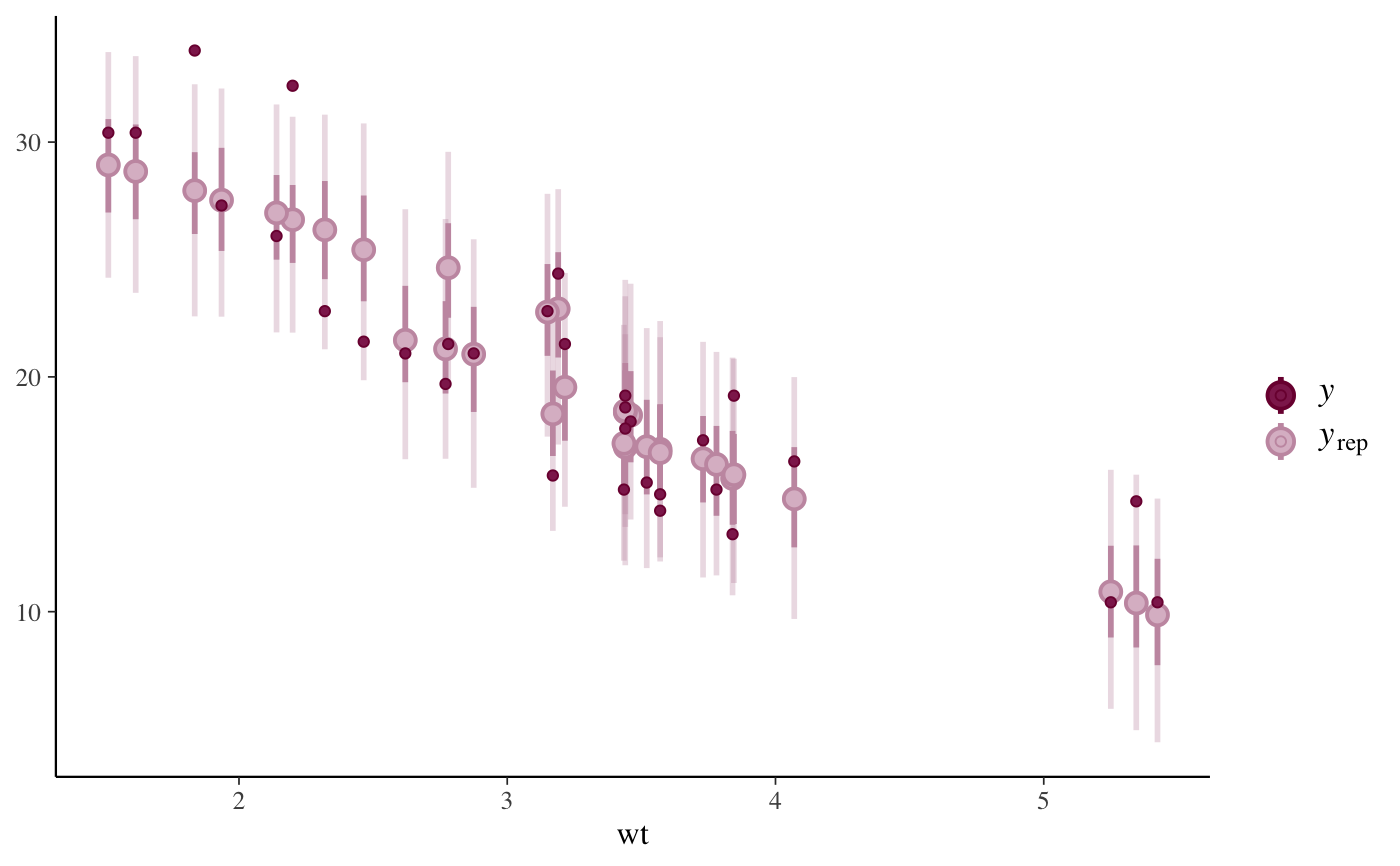# predictive errors pp_check(fit, plotfun = "error_hist", nreps = 6)#> stat_bin() using bins = 30. Pick better value with binwidth.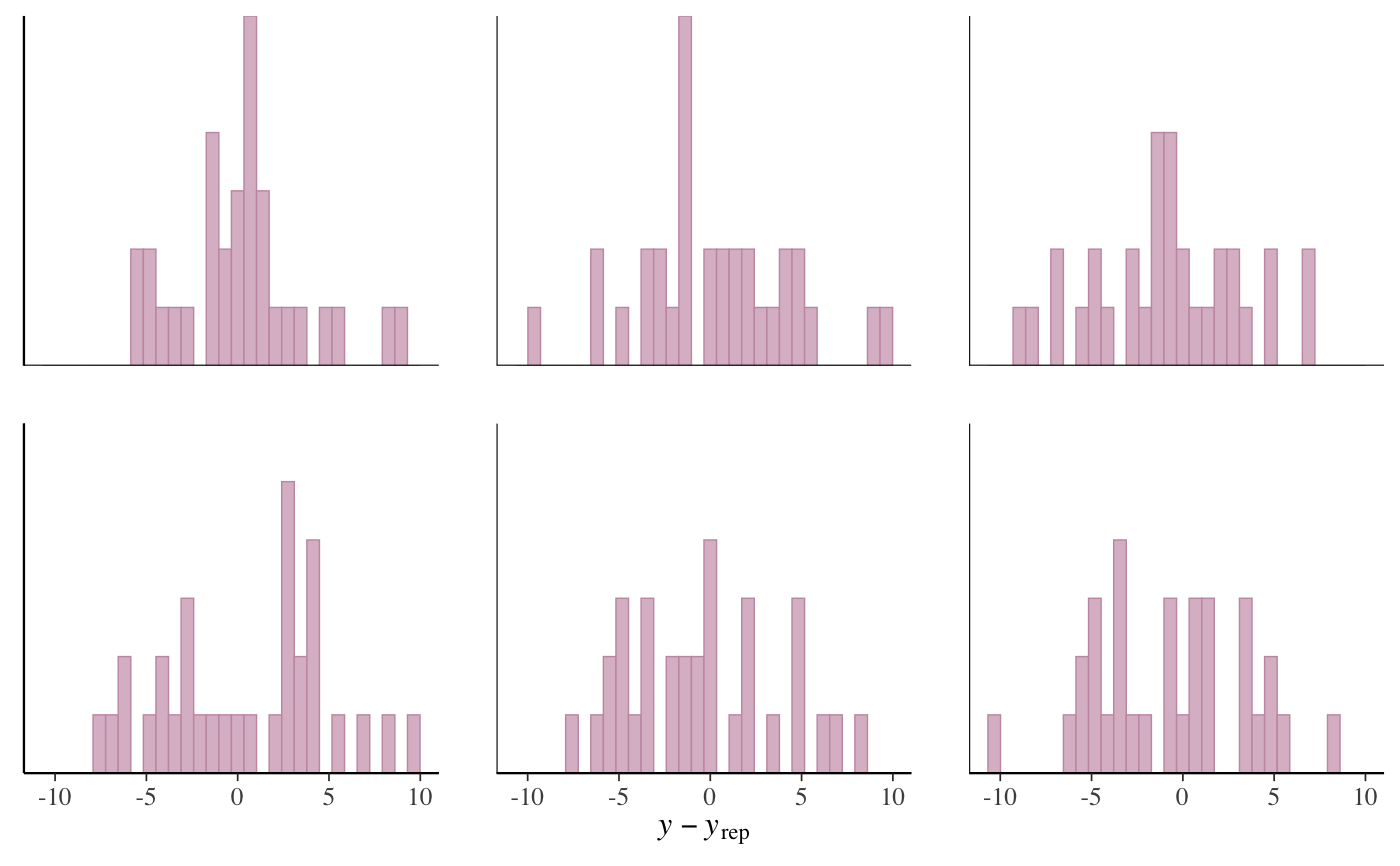pp_check(fit, plotfun = "error_scatter_avg_vs_x", x = "wt") + ggplot2::xlab("wt")# Example of a PPC for ordinal models (stan_polr) fit2 <- stan_polr(tobgp ~ agegp, data = esoph, method = "probit", prior = R2(0.2, "mean"), init_r = 0.1, refresh = 0) pp_check(fit2, plotfun = "bars", nreps = 500, prob = 0.5)pp_check(fit2, plotfun = "bars_grouped", group = esoph$agegp,
nreps = 500, prob = 0.5)# }